2 sin x sin y formula

Chapter 3 Class 11 Trigonometric Functions
Concept wise

In Trigonometry Formulas, we will learn

## Basic Formulas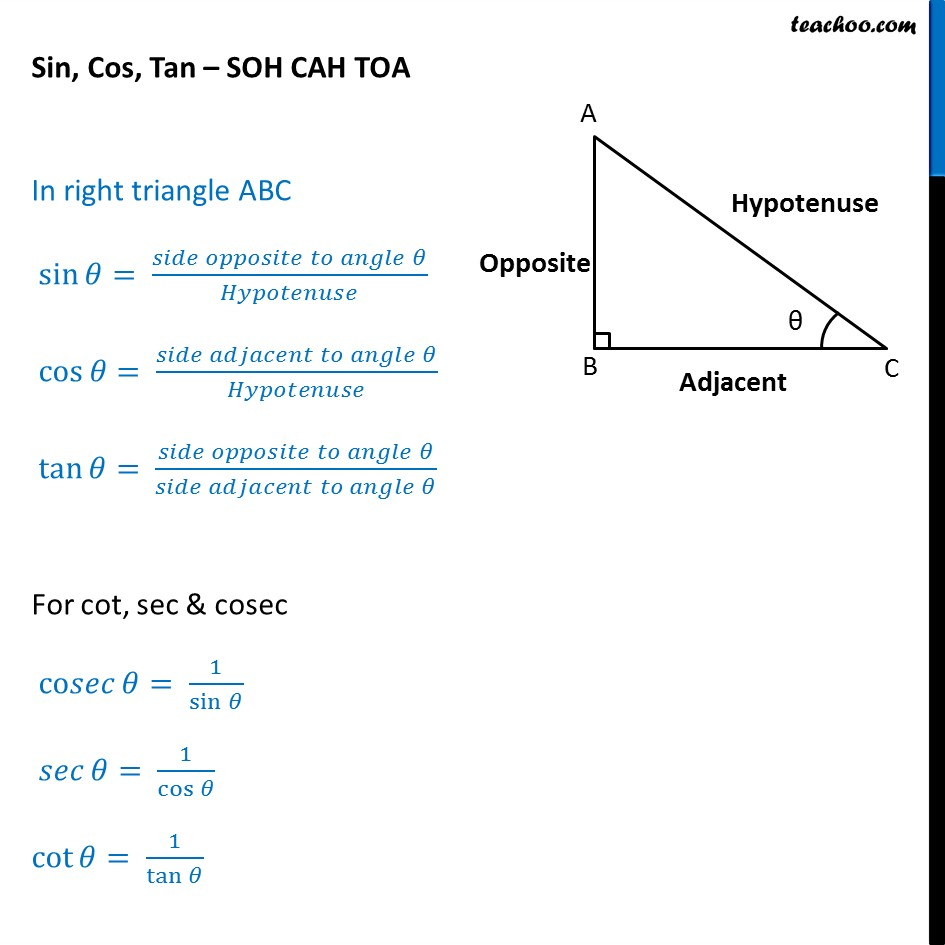## sin, cos tan at 0, 30, 45, 60 degrees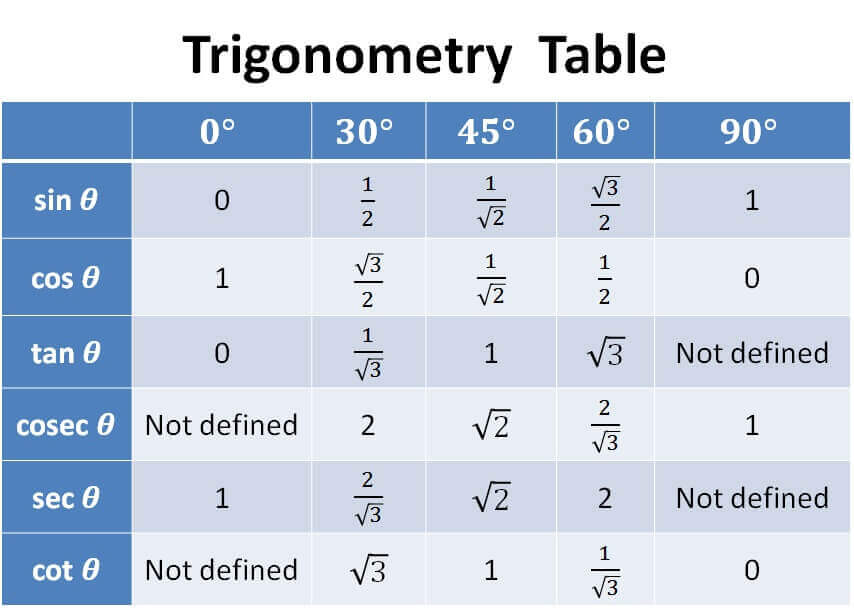## Pythagorean Identities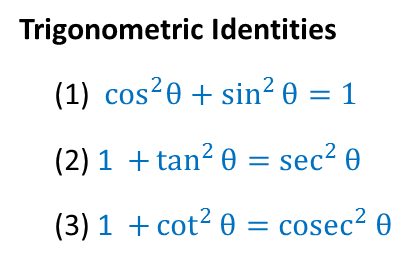## Signs of sin, cos, tan in different quadrants

To learn sign of sin, cos, tan in different quadrants,

we remember

A dd → S ugar → T o → C offee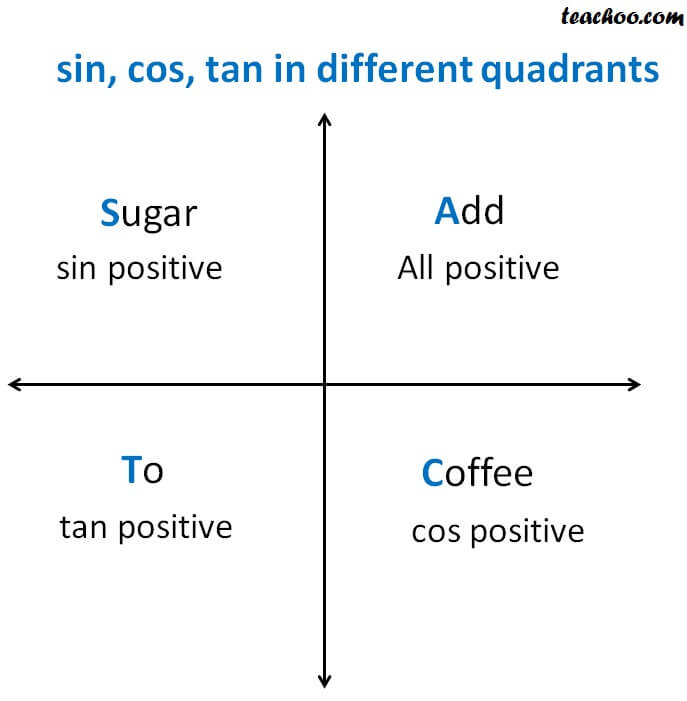Representing as a table

 Quadrant I Quadrant II Quadrant III Quadrant IV sin + + – – cos + – – – tan + – + –

Radian measure = π/180  ×  Degree measure

Also,

1 Degree = 60 minutes

i.e. 1° = 60’

1 Minute = 60 seconds

i.e. 1’ = 60’’

## Negative angles (Even-Odd Identities)

sin (–x) = – sin x

cos (–x) = cos x

tan (–x) = – tan x

sec (–x) = sec x

cosec (–x) = – cosec x

cot (–x) = – cot x

## Value of sin, cos, tan repeats after 2π

sin (2π + x) = sin x

cos (2π + x) = cos x

tan (2π + x) = tan x

## Shifting angle by π/2, π,  3π/2 (Co-Function Identities or Periodicity Identities)

 sin (π/2 – x) = cos x cos (π/2 – x) = sin x sin (π/2 + x) = cos x cos (π/2 + x) = – sin x sin (3π/2 – x)  = – cos x cos (3π/2 – x)  = – sin x sin (3π/2 + x) = – cos x cos (3π/2 + x) = sin x sin (π – x) = sin x cos (π – x) = – cos x sin (π + x) = – sin x cos (π + x) = – cos x sin (2π – x) = – sin x cos (2π – x) = cos x sin (2π + x) = sin x cos (2π + x) = cos x

## Angle sum and difference identities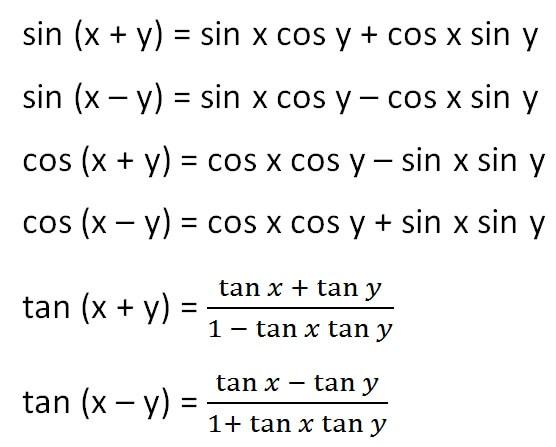## Double Angle Formulas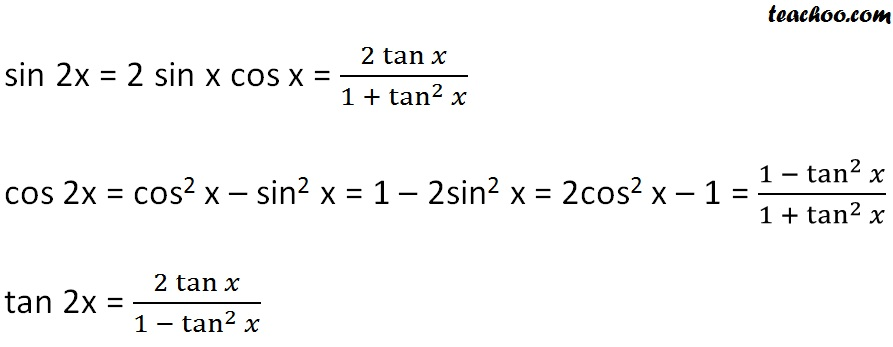## Triple Angle Formulas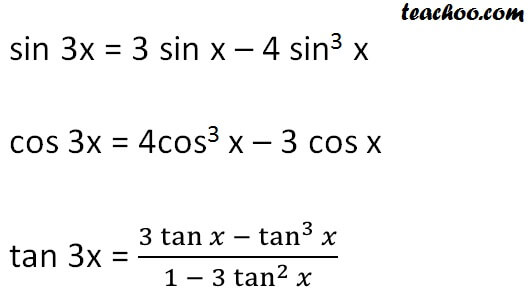## Half Angle Identities (Power reducing formulas)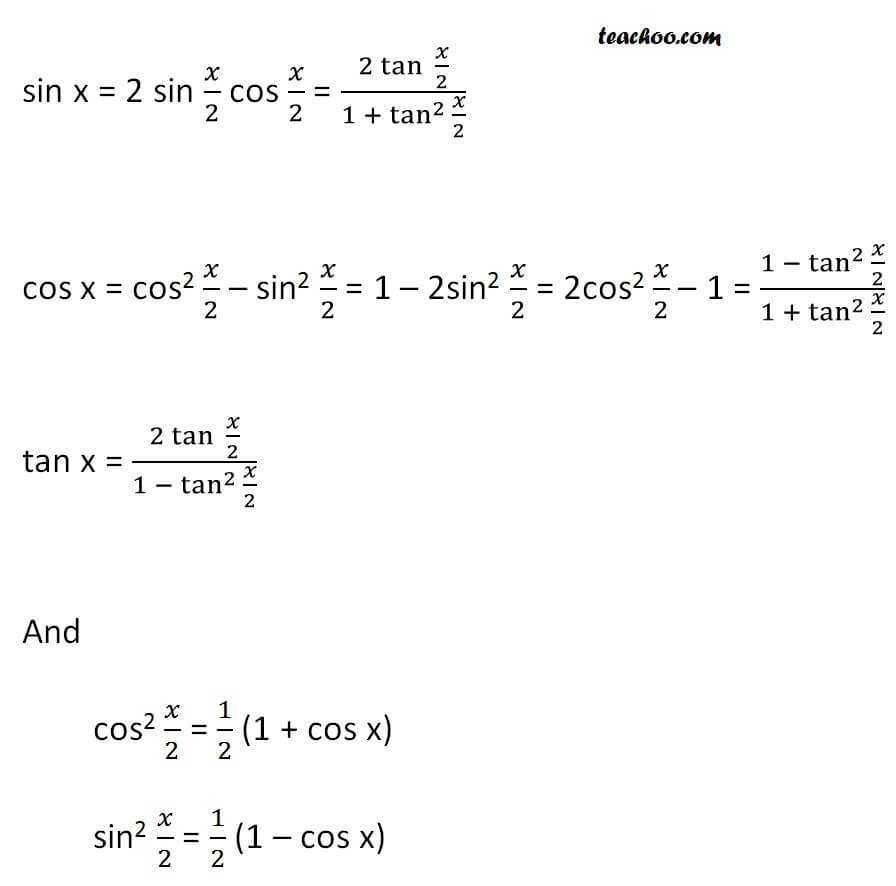## Sum Identities (Sum to Product Identities)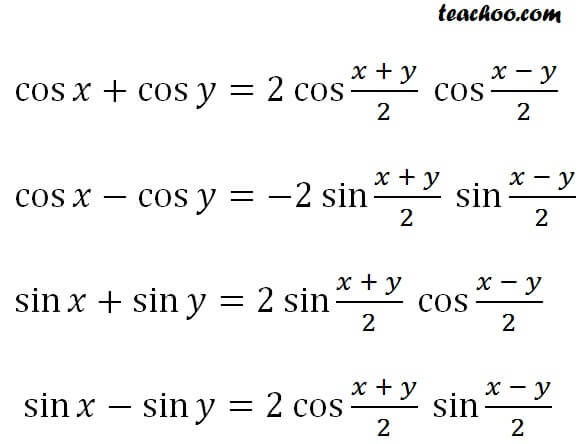## Product Identities (Product to Sum Identities)

Product to sum identities are

2 cos⁡x  cos⁡y = cos⁡ (x + y) + cos⁡(x - y)

-2 sin⁡x  sin⁡y = cos⁡ (x + y) - cos⁡(x - y)

2 sin⁡x  cos⁡y = sin⁡ (x + y) + sin⁡(x - y)

2 cos⁡x  sin⁡y = sin⁡ (x + y) - sin⁡(x - y)

## Law of sine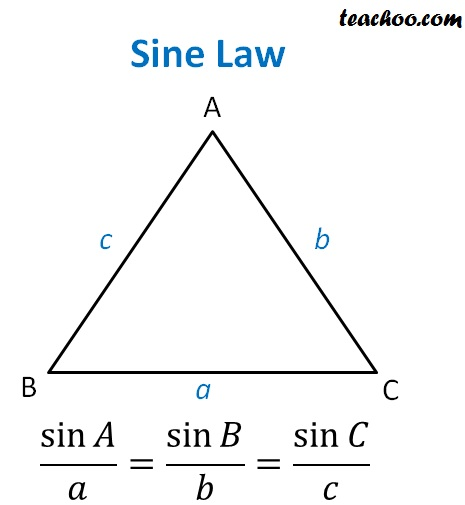Here

• A, B, C are vertices of Δ ABC
• a is side opposite to A i.e. BC
• b is side opposite to B i.e. AC
• c is side opposite to C i.e. AB

## Law of cosine

Just like Sine Law, we have cosine Law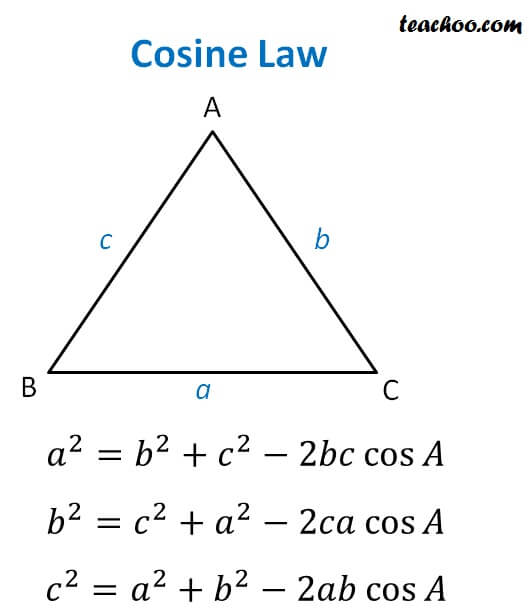## What are Inverse Trigonometric Functions

If sin θ = x

Then putting sin on the right side

θ = sin -1 x

sin -1 x = θ

So, inverse of sin is an angle.

Similarly, inverse of all the trigonometry function is angle.

Note : Here angle is measured in radians, not degrees.

So, we have

sin -1 x

cos -1 x

tan -1 x

cosec -1 x

sec -1 x

tan -1 x

## Domain and Range of Inverse Trigonometric Functions

 Domain Range sin -1 [–1, 1] [-π/2,π/2] cos -1 [–1, 1] [0,π] tan -1 R (-π/2,π/2) cosec -1 R – (–1, 1) [π/2,π/2] - {0} sec -1 R – (–1, 1) [0,π]-{π/2} cot -1 R (0,π)

## Some formulae for Inverse Trigonometry are

sin –1 (–x) = – sin -1 x

cos –1 (–x) = π – sin -1 x

tan –1 (–x) = – tan -1 x

cosec –1 (–x) = – cosec -1 x

sec –1 (–x) = – sec -1 x

cot –1 (–x) = π – cot -1 x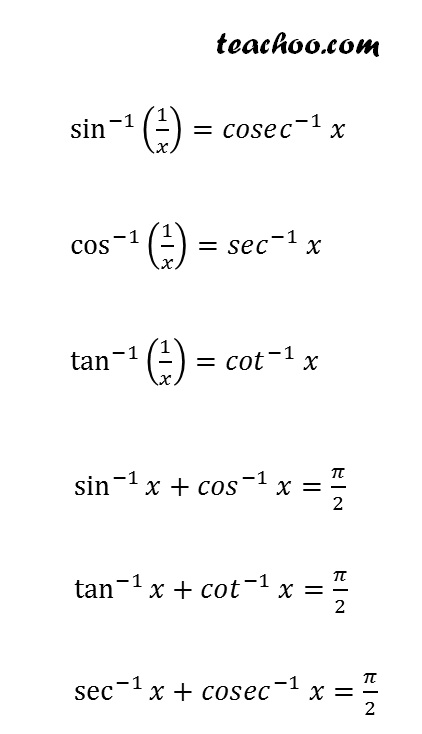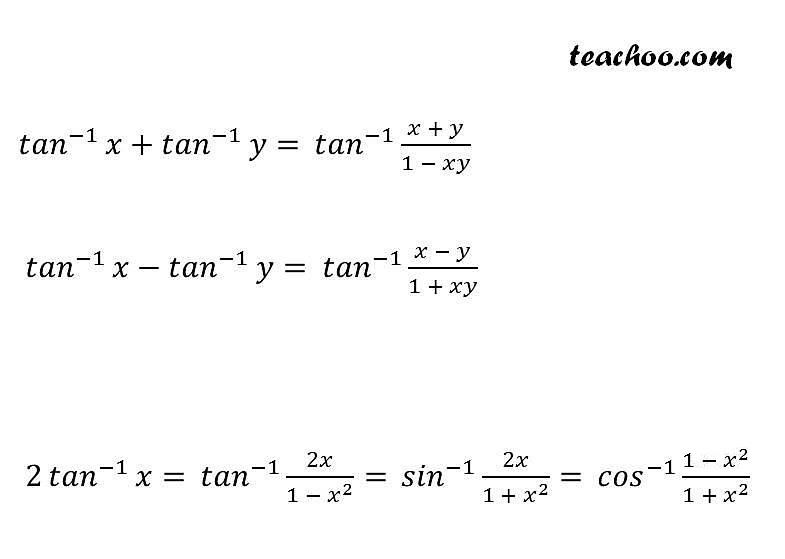## Inverse Trigonometry Substitution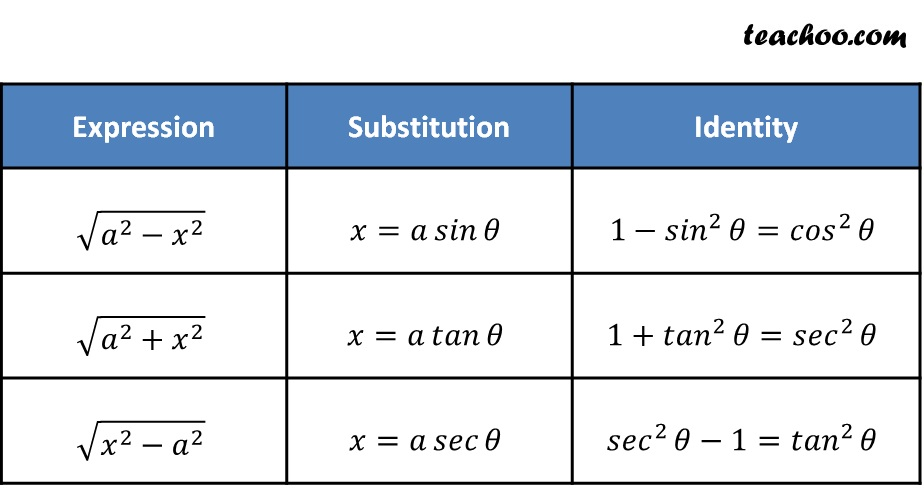Learn in your speed, with individual attention - Teachoo Maths 1-on-1 Class